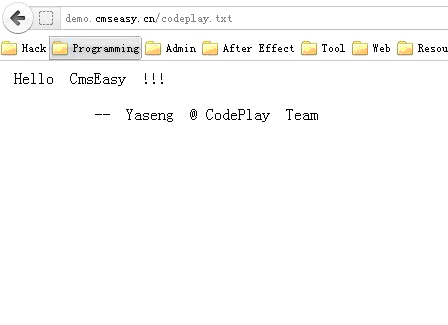Author:Yaseng

Team:CodePlay

```function edit_action() {
if(front::post('submit')) {
\$this->_user->rec_update(front::\$post,'userid='.\$this->view->user['userid']);
front::flash(lang('修改资料成功！'));
front::redirect(url::create('user/index'));
}
\$this->view->data=\$this->view->user;
}```

```function rec_update(\$row,\$where) {
\$tbname=\$this->name;
\$sql=\$this->sql_update(\$tbname,\$row,\$where);

return \$this->query_unbuffered(\$sql);
}```

```function sql_update(\$tbname,\$row,\$where) {
\$sqlud='';
if (is_string(\$row))
\$sqlud=\$row.' ';
else
foreach (\$row as \$key=>\$value) {
if (in_array(\$key,explode(',',\$this->getcolslist()))) {
if (preg_match('/^\[(.*)\]\$/',\$value,\$match))
\$sqlud .= "`\$key`"."= ".\$match.",";
elseif (\$value === "")
\$sqlud .= "`\$key`= NULL, ";
else
\$sqlud .= "`\$key`"."= '".\$value."',";
}
}
\$sqlud=rtrim(\$sqlud);
\$sqlud=rtrim(\$sqlud,',');
\$this->condition(\$where);
\$sql="UPDATE `".\$tbname."` SET ".\$sqlud." WHERE ".\$where;
return \$sql;
}```5 -16  看了一下官方补丁

CodePlay 代码审计  交流群   209547366   （共同进步）